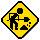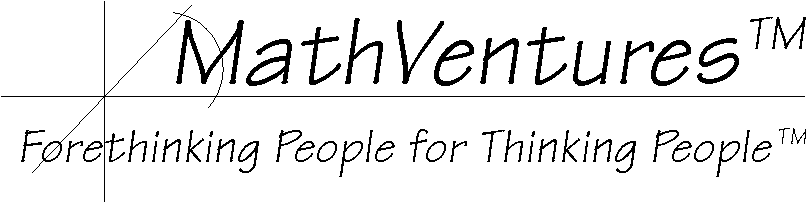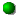Hard hat area, construction in progress# MathEd

### Passionate About Math EducationThe Numbers

####Algebra

All of the documents that are available for you to download are published using Adobe Acrobat format (.PDF). You must use Adobe Acrobat Reader in order to view these files. If you don't have a copy of Adobe Acrobat Reader, it is available for free at CNet.com*, or ZDNet.com* where you should click on Downloads, if you are not already there, and then search for Acrobat, limiting your search to In Downloads, if this option is available.

*   More direct links cannot not be given here because these sites are changed frequently.
These links open is a separate window so you do not lose your place here.

No borrowing, no negative numbers, this simple method makes subtraction easy.
The standard multi-digit subtraction procedure (algorithm) that is taught to most, if not all, students requires that the student is capable of subtracting any single-digit number from any number in the range of 10 to 19. For the student must be able to "borrow" a "ten" from the digit to the left of the current one. In other words, the strongest demand on the student's mathematical skill is the ability to mentally subtract any single digit number from any number between 10 and 19, inclusively.

For the students who struggle with this requirement this new procedure provides an easier alternative. It required that the strongest subtraction skill is the ability to subtract any single-digit number from any single-digit number. It also requires the ability to add multi-digit numbers, a skill that students are assumed to possess before they are taught multi-digit subtraction.

This procedure does not employ borrowing. Instead it replaces the source number with a substitute from which the subtraction can proceed without borrowing. After the subtraction is finished, the result is adjusted by adding the number that was used to generate the substitute source number.

A bonus of this procedure is the fact the actual subtraction can proceed from left to right just as traditionally it is done from right to left. In fact, as long as digits are subtracted in their columns and subtraction is carried out in each and every column, the order is no longer relevant.How to Find the Greatest Common Factor (GCF), Visually and Without any Calculation

This graphic method is completely visual and requires no computation. It makes use of relationship between geometry and arithmatic.

When given two integers, mandn, the common method to find their GCF is to divide both numbers by the most obvious factors until all are exhausted. Then GCF is equal to the product of all of these factors. For example, if both mandn are even divide them by 2. If the results m1 and n1are again even, divide again by 2. If other obvious factors are known, such as 3, 5, 9 or 11, then divide by these factors. The problem is that often no factor is easily apparent for both m and n.

This alternative method does not rely on knowing any common factors. It employs simple division and each division step is progressively simpler. Or, you may even use this method without ever using division. Instead you may choose to only use subtraction.

Also, this method has graphical representation. In other words, this method facilitate finding the greatest common factor without doing any calculation  you only need to count  instead you draw and the solution appears. When teaching visually-oriented students, who are experiencing difficulties understanding the computational method, employing this graphic method, may be easier.

To see animated examples click Find Greatest Common Factor, Examples.